x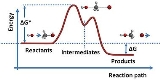Electromotive forceOverview

In physics
Physics
Physics is a natural science that involves the study of matter and its motion through spacetime, along with related concepts such as energy and force. More broadly, it is the general analysis of nature, conducted in order to understand how the universe behaves.Physics is one of the oldest academic...

, electromotive force, emf (seldom capitalized), or electromotance (denoted ε and measured in volts) refers to voltage
Voltage
Voltage, otherwise known as electrical potential difference or electric tension is the difference in electric potential between two points — or the difference in electric potential energy per unit charge between two points...

generated by a battery
Battery (electricity)
An electrical battery is one or more electrochemical cells that convert stored chemical energy into electrical energy. Since the invention of the first battery in 1800 by Alessandro Volta and especially since the technically improved Daniell cell in 1836, batteries have become a common power...

or by the magnetic force according to Faraday's Law
Faraday's law of induction dates from the 1830s, and is a basic law of electromagnetism relating to the operating principles of transformers, inductors, and many types of electrical motors and generators...

, which states that a time varying magnetic field
Magnetic field
A magnetic field is a mathematical description of the magnetic influence of electric currents and magnetic materials. The magnetic field at any given point is specified by both a direction and a magnitude ; as such it is a vector field.Technically, a magnetic field is a pseudo vector;...

will induce an electric current
Electric current
Electric current is a flow of electric charge through a medium.This charge is typically carried by moving electrons in a conductor such as wire...

.

It is important to note that the electromotive "force" is not a force
Force
In physics, a force is any influence that causes an object to undergo a change in speed, a change in direction, or a change in shape. In other words, a force is that which can cause an object with mass to change its velocity , i.e., to accelerate, or which can cause a flexible object to deform...

in the classical physics sense—as can be seen in the fact that it is measured in volts and not newtons.Describe the relationship between e.m.f and p.d in a single loop circuitWhy emf can not be zero while potential difference can be zero? What is difference in both of them?Encyclopedia
In physics
Physics
Physics is a natural science that involves the study of matter and its motion through spacetime, along with related concepts such as energy and force. More broadly, it is the general analysis of nature, conducted in order to understand how the universe behaves.Physics is one of the oldest academic...

, electromotive force, emf (seldom capitalized), or electromotance (denoted ε and measured in volts) refers to voltage
Voltage
Voltage, otherwise known as electrical potential difference or electric tension is the difference in electric potential between two points — or the difference in electric potential energy per unit charge between two points...

generated by a battery
Battery (electricity)
An electrical battery is one or more electrochemical cells that convert stored chemical energy into electrical energy. Since the invention of the first battery in 1800 by Alessandro Volta and especially since the technically improved Daniell cell in 1836, batteries have become a common power...

or by the magnetic force according to Faraday's Law
Faraday's law of induction dates from the 1830s, and is a basic law of electromagnetism relating to the operating principles of transformers, inductors, and many types of electrical motors and generators...

, which states that a time varying magnetic field
Magnetic field
A magnetic field is a mathematical description of the magnetic influence of electric currents and magnetic materials. The magnetic field at any given point is specified by both a direction and a magnitude ; as such it is a vector field.Technically, a magnetic field is a pseudo vector;...

will induce an electric current
Electric current
Electric current is a flow of electric charge through a medium.This charge is typically carried by moving electrons in a conductor such as wire...

.

It is important to note that the electromotive "force" is not a force
Force
In physics, a force is any influence that causes an object to undergo a change in speed, a change in direction, or a change in shape. In other words, a force is that which can cause an object with mass to change its velocity , i.e., to accelerate, or which can cause a flexible object to deform...

in the classical physics sense—as can be seen in the fact that it is measured in volts and not newtons. Formally, emf is the external work expended per unit of charge
Electric charge
Electric charge is a physical property of matter that causes it to experience a force when near other electrically charged matter. Electric charge comes in two types, called positive and negative. Two positively charged substances, or objects, experience a mutual repulsive force, as do two...

to produce an electric potential difference across two open-circuited terminals.
The electric potential difference produced is created by separating positive and negative charges, thereby generating an electric field. The created electrical potential difference drives current flow if a circuit is attached to the source of emf. When current flows, however, the voltage across the terminals of the source of emf is no longer the open-circuit value, due to voltage drops inside the device due to its internal resistance
Internal resistance
A practical electrical power source which is a linear electric circuit may, according to Thévenin's theorem, be represented as an ideal voltage source in series with an impedance. This resistance is termed the internal resistance of the source. When the power source delivers current, the measured...

.

Devices that can provide emf include electrochemical cell
Electrochemical cell
An electrochemical cell is a device capable of either deriving electrical energy from chemical reactions, or facilitating chemical reactions through the introduction of electrical energy. A common example of an electrochemical cell is a standard 1.5-volt "battery"...

s, thermoelectric device
Thermoelectric effect
The thermoelectric effect is the direct conversion of temperature differences to electric voltage and vice-versa. A thermoelectric device creates a voltage when there is a different temperature on each side. Conversely, when a voltage is applied to it, it creates a temperature difference...

s, solar cells, electrical generator
Electrical generator
In electricity generation, an electric generator is a device that converts mechanical energy to electrical energy. A generator forces electric charge to flow through an external electrical circuit. It is analogous to a water pump, which causes water to flow...

s, transformer
Transformer
A transformer is a device that transfers electrical energy from one circuit to another through inductively coupled conductors—the transformer's coils. A varying current in the first or primary winding creates a varying magnetic flux in the transformer's core and thus a varying magnetic field...

s, and even Van de Graaff generator
Van de Graaff generator
A Van de Graaff generator is an electrostatic generator which uses a moving belt to accumulate very high voltages on a hollow metal globe on the top of the stand. It was invented in 1929 by American physicist Robert J. Van de Graaff. The potential differences achieved in modern Van de Graaff...

s. In nature, emf is generated whenever magnetic field fluctuations occur through a surface. An example for this is the varying Earth magnetic field during a geomagnetic storm
Geomagnetic storm
A geomagnetic storm is a temporary disturbance of the Earth's magnetosphere caused by a disturbance in the interplanetary medium. A geomagnetic storm is a major component of space weather and provides the input for many other components of space weather...

, acting on anything on the surface of the planet, like an extended electrical grid.

In the case of a battery, charge separation that gives rise to a voltage difference is accomplished by chemical reactions at the electrodes; a voltaic cell can be thought of as having a "charge pump" of atomic dimensions at each electrode, that is:

A source of emf can be thought of as a kind of charge pump that acts to move positive charge from a point of low potential through its interior to a point of high potential. … By chemical, mechanical or other means, the source of emf performs work dW on that charge to move it to the high potential terminal. The emf of the source is defined as the work dW done per charge dq: = dW/dq.

Around 1830 Faraday established that the reactions at each of the two electrode–electrolyte interfaces provide the "seat of emf" for the voltaic cell, that is, these reactions drive the current. In the open-circuit case, charge separation continues until the electrical field from the separated charges is sufficient to arrest the reactions. Years earlier, Volta, who had measured a contact potential difference at the metal-metal (electrode-electrode) interface of his cells, held the incorrect opinion that this contact potential was the origin of the seat of emf.

In the case of an electrical generator, a time-varying magnetic field inside the generator creates an electric field via electromagnetic induction
Electromagnetic induction
Electromagnetic induction is the production of an electric current across a conductor moving through a magnetic field. It underlies the operation of generators, transformers, induction motors, electric motors, synchronous motors, and solenoids....

, which in turn creates an energy difference between generator terminals. Charge separation takes place within the generator, with electrons flowing away from one terminal and toward the other, until, in the open-circuit case, sufficient electric field builds up to make further movement unfavorable. Again the emf is countered by the electrical voltage due to charge separation. If a load is attached, this voltage can drive a current. The general principle governing the emf in such electrical machines is Faraday's law of induction
Faraday's law of induction dates from the 1830s, and is a basic law of electromagnetism relating to the operating principles of transformers, inductors, and many types of electrical motors and generators...

.

A solar cell
Solar cell
A solar cell is a solid state electrical device that converts the energy of light directly into electricity by the photovoltaic effect....

or photodiode
Photodiode
A photodiode is a type of photodetector capable of converting light into either current or voltage, depending upon the mode of operation.The common, traditional solar cell used to generateelectric solar power is a large area photodiode....

is another source of emf, with light energy as the external power source.

## Notation and units of measurement

Electromotive force is often denoted by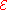or (script capital E, Unicode U+2130).

In a device without internal resistance, if an electric charge
Electric charge
Electric charge is a physical property of matter that causes it to experience a force when near other electrically charged matter. Electric charge comes in two types, called positive and negative. Two positively charged substances, or objects, experience a mutual repulsive force, as do two...

Q passes through that device, and gains an energy
Energy
In physics, energy is an indirectly observed quantity. It is often understood as the ability a physical system has to do work on other physical systems...

W, the net emf for that device is the energy gained per unit charge
Electric charge
Electric charge is a physical property of matter that causes it to experience a force when near other electrically charged matter. Electric charge comes in two types, called positive and negative. Two positively charged substances, or objects, experience a mutual repulsive force, as do two...

, or W/Q. Like other measures of energy per charge, emf has SI
International System of Units
The International System of Units is the modern form of the metric system and is generally a system of units of measurement devised around seven base units and the convenience of the number ten. The older metric system included several groups of units...

units of volt
Volt
The volt is the SI derived unit for electric potential, electric potential difference, and electromotive force. The volt is named in honor of the Italian physicist Alessandro Volta , who invented the voltaic pile, possibly the first chemical battery.- Definition :A single volt is defined as the...

s, equivalent to joule
Joule
The joule ; symbol J) is a derived unit of energy or work in the International System of Units. It is equal to the energy expended in applying a force of one newton through a distance of one metre , or in passing an electric current of one ampere through a resistance of one ohm for one second...

s per coulomb.

Electromotive force in electrostatic units is the statvolt
Statvolt
The statvolt is a unit of voltage and electrical potential used in the cgs system of units. The conversion to the SI system isIt is a useful unit for electromagnetism because one statvolt per centimetre is equal in magnitude to one gauss. Thus, for example, an electric field of one statvolt/cm has...

(in the centimeter gram second system of units equal in amount to an erg
Erg
An erg is the unit of energy and mechanical work in the centimetre-gram-second system of units, symbol "erg". Its name is derived from the Greek ergon, meaning "work"....

per electrostatic unit of charge
Electric charge
Electric charge is a physical property of matter that causes it to experience a force when near other electrically charged matter. Electric charge comes in two types, called positive and negative. Two positively charged substances, or objects, experience a mutual repulsive force, as do two...

).

## Terminology

The term electromotive force is due to Alessandro Volta
Alessandro Volta
Count Alessandro Giuseppe Antonio Anastasio Gerolamo Umberto Volta was a Lombard physicist known especially for the invention of the battery in 1800.-Early life and works:...

(1745–1827), who invented the battery, or voltaic pile
Voltaic pile
A voltaic pile is a set of individual Galvanic cells placed in series. The voltaic pile, invented by Alessandro Volta in 1800, was the first electric battery...

. "Electromotive force" originally referred to the 'force
Force
In physics, a force is any influence that causes an object to undergo a change in speed, a change in direction, or a change in shape. In other words, a force is that which can cause an object with mass to change its velocity , i.e., to accelerate, or which can cause a flexible object to deform...

' with which positive and negative charges could be separated (that is, moved, hence "electromotive"), and was also called "electromotive power" (although it is not a power
Power (physics)
In physics, power is the rate at which energy is transferred, used, or transformed. For example, the rate at which a light bulb transforms electrical energy into heat and light is measured in watts—the more wattage, the more power, or equivalently the more electrical energy is used per unit...

in the modern sense). Maxwell's 1865 explanation of what are now called Maxwell's equations
Maxwell's equations
Maxwell's equations are a set of partial differential equations that, together with the Lorentz force law, form the foundation of classical electrodynamics, classical optics, and electric circuits. These fields in turn underlie modern electrical and communications technologies.Maxwell's equations...

used the term "electromotive force" for what is now called the electric field
Electric field
In physics, an electric field surrounds electrically charged particles and time-varying magnetic fields. The electric field depicts the force exerted on other electrically charged objects by the electrically charged particle the field is surrounding...

strength.
But, in his later textbook
he uses the term "electromotive force" both for "voltage-like" causes of current flow in an electric circuit, and (inconsistently) for contact potential difference (which is a form of electrostatic potential difference
Electric potential
In classical electromagnetism, the electric potential at a point within a defined space is equal to the electric potential energy at that location divided by the charge there...

). Given that Maxwell's textbook was written before the discovery of the electron, it is understandable that Maxwell exhibits what (in terms of modern knowledge) is inconsistency in the use of the term "electromotive force".

The word "force" in "electromotive force" is a misnomer:

[Electromotive force] has turned out to be an unfortunate choice of words which is still with us 160 years later. In all of physics except electromagnetic induction, the term 'force' is reserved for mechanical action on ponderable matter and is measured in units called newtons. In contrast electromotive force is measured in units of volts and causes charge separation.

Nonetheless, the term "electromotive force" has resisted change. "Electromotance", meaning (literally) tendency to move ("-motance") electrical charge, is semantically more accurate, but not widely adopted. Both terms are less common than the abbreviation emf.

These terms (emf, voltage, etc.) have many interpretations and applications, not all necessarily consistent with each other. The emf is typically considered to be the work done per unit charge by a source in creating a separation of positive from negative charges, thereby creating a voltage difference; the work done per unit charge in pushing charge through a battery creating the battery's voltage difference, for example. However, there is not complete unanimity upon this usage. As Sydney Ross says, in excusing himself for avoiding the term emf:

We have refrained from using the term 'electromotive force' or 'e.m.f.' for short; for there is no consistency between different authors in the meaning of the term. … To some authors it is synonymous with 'voltage.' To others it means the open-circuit voltage of a battery. To a third group of authors it means the open-circuit voltage of any two-terminal device. This use is met most often in connection with Thevenin's theorem in circuit theory. To a fourth group it means the work accounted for by agencies other than differences of the (not measurable) Galvani potentials. Such authors equate the current–resistance
product of a circuit branch to the sum of voltage plus e.m.f. A fifth
group extends this use to field theory. The authors of this group
equate the product of current density and resistivity to the sum of
electric-field strength plus an e.m.f. gradient. A sixth group applies
the term to electromagnetic induction. These authors define e.m.f.
as the spatial line integral of the electric-field strength taken over
a complete loop. To them the term 'counter e.m.f.' means something.

It is common in some fields, such as circuit theory, to refer to the voltage created by the emf as the emf.
Some authors do not distinguish between the emf and the voltage it creates. Some use emf to refer to the open-circuit voltage and voltage to the potential difference when current is drawn.
Here is a quotation describing emf as an open-circuit voltage difference:
This buildup of charge on the electrodes tends to oppose the current flow with a 'back voltage' ΔV. On an open circuit, I = 0 the value of ΔV for which I = 0 is defined as the emf of the cell. That is, = ΔVI=0.

This usage does not identify the work done per unit charge in creating the charge build-up as emf, but rather identifies emf with the consequent "back voltage" that arrests current flow in the open-circuit condition.
One emphasizes the conversion of energy from other forms to electrical energy, the other emphasizes the resulting electrical potential. This article focuses upon the conversion of other forms of energy to electrical potential energy, and describes some examples of how this conversion comes about.

## Formal definitions of electromotive force

Inside a source of emf that is open-circuited, the conservative electrostatic field created by separation of charge exactly cancels the forces producing the emf. Thus, the emf has the same value but opposite sign as the integral of the electric field
Electric field
In physics, an electric field surrounds electrically charged particles and time-varying magnetic fields. The electric field depicts the force exerted on other electrically charged objects by the electrically charged particle the field is surrounding...

aligned with an internal path between two terminals A and B of a source of emf in open-circuit condition (the path is taken from the negative terminal to the positive terminal to yield a positive emf, indicating work done on the electrons moving in the circuit).
Mathematically: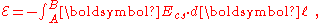where Ecs is the conservative electrostatic field created by the charge separation associated with the emf, dℓ is an element of the path from terminal A to terminal B, and ‘·’ denotes the vector dot product
Dot product
In mathematics, the dot product or scalar product is an algebraic operation that takes two equal-length sequences of numbers and returns a single number obtained by multiplying corresponding entries and then summing those products...

. This equation applies only to locations
A and B that are terminals, and does not apply to paths between points A and B with portions outside the source of emf. This equation involves the electrostatic electric field due to charge separation Ecs and does not involve (for example) any non-conservative component of electric field due to Faraday's law of induction.

In the case of a closed path in the presence of a varying magnetic field
Magnetic field
A magnetic field is a mathematical description of the magnetic influence of electric currents and magnetic materials. The magnetic field at any given point is specified by both a direction and a magnitude ; as such it is a vector field.Technically, a magnetic field is a pseudo vector;...

, the integral of the electric field
Electric field
In physics, an electric field surrounds electrically charged particles and time-varying magnetic fields. The electric field depicts the force exerted on other electrically charged objects by the electrically charged particle the field is surrounding...

around a closed loop may be nonzero; one common application of the concept of emf, known as "induced emf" is the voltage induced in a such a loop. The "induced emf" around a stationary closed path C is: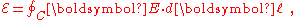where now E is the entire electric field, conservative and non-conservative, and the integral is around an arbitrary but stationary closed curve
C through which there is a varying magnetic field. Note that the electrostatic field does not contribute to the net emf around a circuit because the electrostatic portion of the electric field is conservative
Conservative force
A conservative force is a force with the property that the work done in moving a particle between two points is independent of the path taken. Equivalently, if a particle travels in a closed loop, the net work done by a conservative force is zero.It is possible to define a numerical value of...

(that is, the work done against the field around a closed path is zero).

This definition can be extended to arbitrary sources of emf and moving paths
C: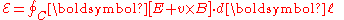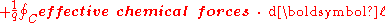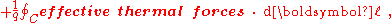which is a conceptual equation mainly, because the determination of the "effective forces" is difficult.

## Electromotive force in thermodynamics

When multiplied by an amount of charge dZ the emf ℰ yields a thermodynamic work term ℰdZ that is used in the formalism for the change in Gibbs free energy
Gibbs free energy
In thermodynamics, the Gibbs free energy is a thermodynamic potential that measures the "useful" or process-initiating work obtainable from a thermodynamic system at a constant temperature and pressure...

when charge is passed in a battery: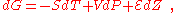where
G is the Gibb's free energy, S is the entropy
Entropy
Entropy is a thermodynamic property that can be used to determine the energy available for useful work in a thermodynamic process, such as in energy conversion devices, engines, or machines. Such devices can only be driven by convertible energy, and have a theoretical maximum efficiency when...

,
V is the system volume, P is its pressure and T is its absolute temperature.

The combination ( ℰ,
Z ) is an example of a conjugate pair of variables
Conjugate variables (thermodynamics)
In thermodynamics, the internal energy of a system is expressed in terms of pairs of conjugate variables such as temperature/entropy or pressure/volume. In fact all thermodynamic potentials are expressed in terms of conjugate pairs....

. At constant pressure the above relationship produces a Maxwell relation that links the change in open cell voltage with temperature
T (a measurable quantity) to the change in entropy S when charge is passed isothermally and isobarically. The latter is closely related to the reaction entropy
Entropy
Entropy is a thermodynamic property that can be used to determine the energy available for useful work in a thermodynamic process, such as in energy conversion devices, engines, or machines. Such devices can only be driven by convertible energy, and have a theoretical maximum efficiency when...

of the electrochemical reaction that lends the battery its power. This Maxwell relation is: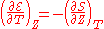If a mole of ions goes into solution (for example, in a Daniell cell, as discussed below) the charge through the external circuit is: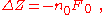where n0 is the number of electrons/ion, and F0 is the Faraday constant and the minus sign indicates discharge of the cell. Assuming constant pressure and volume, the thermodynamic properties of the cell are related strictly to the behavior of its emf by: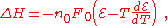where Δ
H is the heat of reaction. The quantities on the right all are directly measurable.

## Electromotive force and voltage difference

An electrical voltage difference is sometimes called an emf. The points below illustrate the more formal usage, in terms of the distinction between emf and the voltage it generates:
1. For a circuit as a whole, such as one containing a resistor in series with a voltaic cell, electrical voltage does not contribute to the overall emf, because the voltage difference on going around a circuit is zero. (The ohmic IR drop plus the applied electrical voltage is zero. See Kirchhoff's Law
Kirchhoff's circuit laws
Kirchhoff's circuit laws are two equalities that deal with the conservation of charge and energy in electrical circuits, and were first described in 1845 by Gustav Kirchhoff...

). The emf is due solely to the chemistry in the battery that causes charge separation, which in turn creates an electrical voltage that drives the current.
2. For a circuit consisting of an electrical generator that drives current through a resistor, the emf is due solely to a time-varying magnetic field that generates an electrical voltage that in turn drives the current. (The ohmic IR drop plus the applied electrical voltage again is zero. See Kirchhoff's Law
Kirchhoff's circuit laws
Kirchhoff's circuit laws are two equalities that deal with the conservation of charge and energy in electrical circuits, and were first described in 1845 by Gustav Kirchhoff...

)
3. A transformer coupling two circuits may be considered a source of emf for one of the circuits, just as if it were caused by an electrical generator; this example illustrates the origin of the term "transformer emf".
4. A photodiode
Photodiode
A photodiode is a type of photodetector capable of converting light into either current or voltage, depending upon the mode of operation.The common, traditional solar cell used to generateelectric solar power is a large area photodiode....

or solar cell
Solar cell
A solar cell is a solid state electrical device that converts the energy of light directly into electricity by the photovoltaic effect....

may be considered as a source of emf, similar to a battery, resulting in an electrical voltage generated by charge separation driven by light rather than chemical reaction.
5. Other devices that produce emf are fuel cell
Fuel cell
A fuel cell is a device that converts the chemical energy from a fuel into electricity through a chemical reaction with oxygen or another oxidizing agent. Hydrogen is the most common fuel, but hydrocarbons such as natural gas and alcohols like methanol are sometimes used...

s, thermocouple
Thermocouple
A thermocouple is a device consisting of two different conductors that produce a voltage proportional to a temperature difference between either end of the pair of conductors. Thermocouples are a widely used type of temperature sensor for measurement and control and can also be used to convert a...

s, and thermopiles.

In the case of an open circuit, the electric charge that has been separated by the mechanism generating the emf creates an electric field opposing the separation mechanism. For example, the chemical reaction in a voltaic cell stops when the opposing electric field at each electrode is strong enough to arrest the reactions. A larger opposing field can reverse the reactions in what are called reversible cells.

The electric charge that has been separated creates an electric potential difference that can be measured with a voltmeter
Voltmeter
A voltmeter is an instrument used for measuring electrical potential difference between two points in an electric circuit. Analog voltmeters move a pointer across a scale in proportion to the voltage of the circuit; digital voltmeters give a numerical display of voltage by use of an analog to...

between the terminals of the device. The magnitude of the emf for the battery (or other source) is the value of this 'open circuit' voltage. When the battery is charging or discharging, the emf itself cannot be measured directly using the external voltage because some voltage is lost inside the source. It can, however, be inferred from a measurement of the current I and voltage difference V, provided that the internal resistance r already has been measured: I = ( − V)/r.

### Chemical sources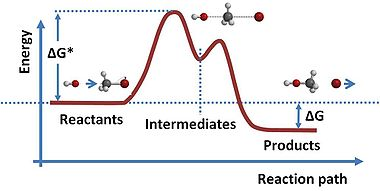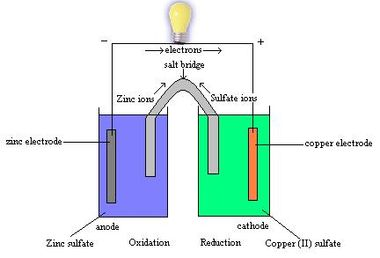The question of how batteries (galvanic cell
Galvanic cell
A Galvanic cell, or Voltaic cell, named after Luigi Galvani, or Alessandro Volta respectively, is an electrochemical cell that derives electrical energy from spontaneous redox reaction taking place within the cell...

s) generate an emf is one that occupied scientists for most of the 19th century. The "seat of the electromotive force" was eventually determined by Walther Nernst
Walther Nernst
Walther Hermann Nernst FRS was a German physical chemist and physicist who is known for his theories behind the calculation of chemical affinity as embodied in the third law of thermodynamics, for which he won the 1920 Nobel Prize in chemistry...

to be primarily at the interfaces between the electrode
Electrode
An electrode is an electrical conductor used to make contact with a nonmetallic part of a circuit...

s and the electrolyte
Electrolyte
In chemistry, an electrolyte is any substance containing free ions that make the substance electrically conductive. The most typical electrolyte is an ionic solution, but molten electrolytes and solid electrolytes are also possible....

.

Molecules are groups of atoms held together by chemical bond
Chemical bond
A chemical bond is an attraction between atoms that allows the formation of chemical substances that contain two or more atoms. The bond is caused by the electromagnetic force attraction between opposite charges, either between electrons and nuclei, or as the result of a dipole attraction...

s, and these bonds consist of electrical forces between electrons (negative) and protons (positive). The molecule in isolation is a stable entity, but when different molecules are brought together, some types of molecules are able to steal electrons from others, resulting in charge separation. This redistribution of charge is accompanied by a change in energy of the system, and a reconfiguration of the atoms in the molecules. The gain of an electron is termed "reduction" and the loss of an electron is termed "oxidation". Reactions in which such electron exchange occurs (which are the basis for batteries) are called reduction-oxidation reactions
Redox
Redox reactions describe all chemical reactions in which atoms have their oxidation state changed....

or redox reactions
Redox
Redox reactions describe all chemical reactions in which atoms have their oxidation state changed....

. In a battery, one electrode is composed of material that gains electrons from the solute, and the other electrode loses electrons, because of these fundamental molecular attributes. The same behavior can be seen in atoms themselves, and their ability to steal electrons is referred to as their electronegativity
Electronegativity
Electronegativity, symbol χ , is a chemical property that describes the tendency of an atom or a functional group to attract electrons towards itself. An atom's electronegativity is affected by both its atomic number and the distance that its valence electrons reside from the charged nucleus...

.

As an example, a Daniell cell
Daniell cell
The Daniell cell was invented in 1836 by John Frederic Daniell, a British chemist and meteorologist, and consisted of a copper pot filled with a copper sulfate solution, in which was immersed an unglazed earthenware container filled with sulfuric acid and a zinc electrode...

consists of a zinc anode (an electron collector), which dissolves into a zinc sulfate solution, the dissolving zinc leaving behind its electrons in the electrode according to the oxidation reaction (s = solid electrode; aq = aqueous solution):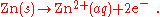The zinc sulfate is an electrolyte
Electrolyte
In chemistry, an electrolyte is any substance containing free ions that make the substance electrically conductive. The most typical electrolyte is an ionic solution, but molten electrolytes and solid electrolytes are also possible....

, that is, a solution in which the components consist of ions, in this case zinc ions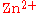, and sulfate ions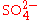.

At the cathode, the copper ions in a copper sulfate electrolyte adopt electrons from the electrode by the reduction reaction: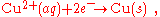and the thus-neutralized copper plates onto the electrode. (A detailed discussion of the microscopic process of electron transfer between an electrode and the ions in an electrolyte may be found in Conway.)

The electrons pass through the external circuit (light bulb in figure), while the ions pass through the salt bridge to maintain charge balance. In the process the zinc anode is dissolved while the copper electrode is plated with copper. If the light bulb is removed (open circuit) the emf between the electrodes is opposed by the electric field due to charge separation, and the reactions stop.

At 273 K, the emf = 1.0934 V, with a temperature coefficient of d/dT = −4.53×10−4 V/K.

#### Voltaic cells

Volta developed the voltaic cell about 1792, and presented his work March 20, 1800. Volta correctly identified the role of dissimilar electrodes in producing the voltage, but incorrectly dismissed any role for the electrolyte. Volta ordered the metals in a 'tension series', “that is to say in an order such that any one in the list becomes positive when in contact with any one that succeeds, but negative by contact with any one that precedes it.” A typical symbolic convention in a schematic of this circuit ( –||– ) would have a long electrode 1 and a short electrode 2, to indicate that electrode 1 dominates. Volta's law about opposing electrode emfs means that, given ten electrodes (for example, zinc and nine other materials), which can be used to produce 45 types of voltaic cells (10 × 9/2), only nine relative measurements (for example, copper and each of the nine others) are needed to get all 45 possible emfs that these ten electrodes can produce.

#### Electromotive force of cells

The electromotive force produced by primary and secondary cells is usually of the order of a few volts. The figures quoted below are nominal, because emf varies according to the size of the load and the state of exhaustion of the cell.
Emf Cell chemistry
1.2 V nickel–metal hydride
1.5 V zinc–carbon
3.6 V to 3.7 V lithium-ion

### Electromagnetic induction

The principle of electromagnetic induction
Electromagnetic induction
Electromagnetic induction is the production of an electric current across a conductor moving through a magnetic field. It underlies the operation of generators, transformers, induction motors, electric motors, synchronous motors, and solenoids....

, noted above, states that a time-dependent magnetic field produces a circulating electric field. A time-dependent magnetic field can be produced either by motion of a magnet relative to a circuit, by motion of a circuit relative to another circuit (at least one of these must be carrying a current), or by changing the current in a fixed circuit. The effect on the circuit itself, of changing the current, is known as self-induction; the effect on another circuit is known as mutual induction.

For a given circuit, the electromagnetically induced emf is determined purely by the rate of change of the magnetic flux through the circuit according to Faraday's law of induction
Faraday's law of induction dates from the 1830s, and is a basic law of electromagnetism relating to the operating principles of transformers, inductors, and many types of electrical motors and generators...

.

An emf is induced in a coil or conductor whenever there is change in the flux linkage
Flux linkage is a property of a coil of conducting wire and the magnetic field through which it passes. It is determined by the number of turns in the coil and the flux of the magnetic field....

s. Depending on the way in which the changes are brought about, there are two types: When the conductor is moved in a stationary magnetic field to procure a change in the flux linkage, the emf is statically induced. The electromotive force generated by motion is often referred to as motional emf. When the change in flux linkage arises from a change in the magnetic field around the stationary conductor, the emf is dynamically induced. The electromotive force generated by a time-varying magnetic field is often referred to as transformer emf.

### Contact potentials

When two different solids are in contact, it is common that thermodynamic equilibrium
Thermodynamic equilibrium
In thermodynamics, a thermodynamic system is said to be in thermodynamic equilibrium when it is in thermal equilibrium, mechanical equilibrium, radiative equilibrium, and chemical equilibrium. The word equilibrium means a state of balance...

requires one of the solids assume a higher electrical potential than the other, the contact potential. For example, dissimilar metals in contact produce what is known also as a contact electromotive force or Galvani potential
Galvani potential
Galvani potential in electrochemistry, is the electric potential difference between two points in the bulk of two phases...

. The magnitude of this potential difference often is expressed as a difference in Fermi level
Fermi level
The Fermi level is a hypothetical level of potential energy for an electron inside a crystalline solid. Occupying such a level would give an electron a potential energy \epsilon equal to its chemical potential \mu as they both appear in the Fermi-Dirac distribution function,which...

s in the two solids, where the Fermi level (a name for the chemical potential
Chemical potential
Chemical potential, symbolized by μ, is a measure first described by the American engineer, chemist and mathematical physicist Josiah Willard Gibbs. It is the potential that a substance has to produce in order to alter a system...

of an electron system) describes the energy necessary to remove an electron from the body. Evidently, if there is an energy advantage in taking an electron from one body to the other, this transfer will occur, thereby causing a charge separation, one body gaining electrons and the other losing electrons. This charge transfer causes a potential difference between the bodies, and therefore, charge transfer becomes more difficult as the charge separation increases. At thermodynamic equilibrium, the gain in energy due to Fermi level difference is matched by the work needed to surmount this potential difference, and at this point no more transfer occurs, and the potential difference has the value called the contact potential. The difference in Fermi levels, on the other hand, is referred to as the emf.
The contact potential cannot drive current through a load attached to its terminals because that current would involve a charge transfer. No mechanism exists to continue such transfer and, hence, maintain a current, once equilibrium is attained.

One might inquire why the contact potential does not appear in Kirchhoff's law of voltages
Kirchhoff's circuit laws
Kirchhoff's circuit laws are two equalities that deal with the conservation of charge and energy in electrical circuits, and were first described in 1845 by Gustav Kirchhoff...

as one contribution to the sum of potential drops. The customary answer is that any circuit involves not only a particular diode or junction, but also all the contact potentials due to wiring and so forth around the entire circuit. The sum of all the contact potentials is zero, and so they may be ignored in Kirchhoff's law.

### Solar cell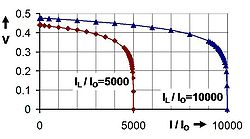Operation of a solar cell can be understood from the equivalent circuit at right. Light, if it includes photons of sufficient energy (greater than the bandgap of the material), creates mobile electron–hole pairs in a semiconductor. Charge separation occurs because of a pre-existing electric field associated with the p-n junction in thermal equilibrium (a contact potential
Volta potential
Volta potential in electrochemistry, is the electric potential difference between two points in the vacuum:...

creates the field). This charge separation between positive hole
Electron hole
An electron hole is the conceptual and mathematical opposite of an electron, useful in the study of physics, chemistry, and electrical engineering. The concept describes the lack of an electron at a position where one could exist in an atom or atomic lattice...

s and negative electron
Electron
The electron is a subatomic particle with a negative elementary electric charge. It has no known components or substructure; in other words, it is generally thought to be an elementary particle. An electron has a mass that is approximately 1/1836 that of the proton...

s across a p-n junction
P-n junction
A p–n junction is formed at the boundary between a P-type and N-type semiconductor created in a single crystal of semiconductor by doping, for example by ion implantation, diffusion of dopants, or by epitaxy .If two separate pieces of material were used, this would...

(a diode
Diode
In electronics, a diode is a type of two-terminal electronic component with a nonlinear current–voltage characteristic. A semiconductor diode, the most common type today, is a crystalline piece of semiconductor material connected to two electrical terminals...

), yields a forward voltage, the photo voltage, between the illuminated diode terminals. As has been noted earlier in the terminology section, the photo voltage is sometimes referred to as the photo emf, rather than distinguishing between the effect and the cause.

The light-induced charge separation creates a reverse current through the cell's junction (that is, not in the direction that a diode normally conducts current), and the charge separation causes a photo voltage that drives current through any attached load. However, a side effect of this voltage is that it tends to forward bias the junction. At high enough levels, this forward bias of the junction will cause a forward current in the diode that subtracts from the current created by the light. Consequently, the greatest current is obtained under short-circuit conditions, and is denoted as IL (for light-induced current) in the equivalent circuit.
Approximately this same current is obtained for forward voltages up to the point where the diode conduction becomes significant.

With this notation, the current-voltage relation for the illuminated diode is: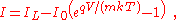where I is the current delivered to the load, I0 is the reverse saturation current, and m the ideality factor, two parameters that depend on the solar cell construction and to some degree upon the voltage itself, and where kT/q is the thermal voltage (about 0.026 V at room temperature). This relation is plotted in the figure using a fixed value m = 2. Under open-circuit conditions (that is, as I → 0), the open-circuit voltage is the voltage at which forward bias of the junction is enough that the forward current completely balances the photocurrent. Rearrangement of the I–V equation provides the open-circuit voltage as: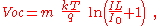which is useful in indicating a logarithmic dependence of Voc upon the light-induced current. Typically, the open-circuit voltage is not more than about 0.5 V.

The value of the photo voltage when driving a load is variable. As shown in the figure, for a load resistance RL, the cell develops a voltage between the short-circuit value V = 0, I = IL and the open-circuit value Voc, I = 0, a value given by Ohm's law
Ohm's law
Ohm's law states that the current through a conductor between two points is directly proportional to the potential difference across the two points...

V = I RL, where the current I is the difference between the short-circuit current and current due to forward bias of the junction, as indicated by the equivalent circuit (neglecting the parasitic resistances).

In contrast to the battery, at current levels near IL, the solar cell acts more like a current source rather than a voltage source.
The current drawn is nearly fixed over a range of load voltages, at one electron per converted photon
Photon
In physics, a photon is an elementary particle, the quantum of the electromagnetic interaction and the basic unit of light and all other forms of electromagnetic radiation. It is also the force carrier for the electromagnetic force...

. The quantum efficiency
Quantum efficiency
Quantum efficiency is a quantity defined for a photosensitive device such as photographic film or a charge-coupled device as the percentage of photons hitting the photoreactive surface that will produce an electron–hole pair. It is an accurate measurement of the device's electrical sensitivity to...

, or probability of getting an electron of photocurrent per incident photon, depends not only upon the solar cell itself, but upon the spectrum of the light.

The diode possesses a "built-in potential" due to the contact potential difference between the two different materials on either side of the junction. This built-in potential is established when the junction is formed as a by-product of thermodynamic equilibrium. Once established, this potential difference cannot drive a current, however, as connecting a load does not upset this equilibrium. In contrast, the accumulation of excess electrons in one region and of excess holes in another due to illumination results in a photo voltage that does drive a current when a load is attached to the illuminated diode. As noted above, this photo voltage also forward biases the junction, and so reduces the pre-existing field in the depletion region
Depletion region
In semiconductor physics, the depletion region, also called depletion layer, depletion zone, junction region or the space charge region, is an insulating region within a conductive, doped semiconductor material where the mobile charge carriers have diffused away, or have been forced away by an...

.

• Counter-electromotive force
Counter-electromotive force
The counter-electromotive force also known as back electromotive force is the voltage, or electromotive force, that pushes against the current which induces it. CEMF is caused by a changing electromagnetic field. It is the effect of Lenz's Law of electromagnetism...

• Electric battery
• Electrochemical cell
Electrochemical cell
An electrochemical cell is a device capable of either deriving electrical energy from chemical reactions, or facilitating chemical reactions through the introduction of electrical energy. A common example of an electrochemical cell is a standard 1.5-volt "battery"...

• Electrolytic cell
Electrolytic cell
An electrolytic cell decomposes chemical compounds by means of electrical energy, in a process called electrolysis; the Greek word lysis means to break up. The result is that the chemical energy is increased...

• Galvanic cell
Galvanic cell
A Galvanic cell, or Voltaic cell, named after Luigi Galvani, or Alessandro Volta respectively, is an electrochemical cell that derives electrical energy from spontaneous redox reaction taking place within the cell...

• Voltaic pile
Voltaic pile
A voltaic pile is a set of individual Galvanic cells placed in series. The voltaic pile, invented by Alessandro Volta in 1800, was the first electric battery...

• Andrew Gray, "Absolute Measurements in Electricity and Magnetism", Electromotive force. Macmillan and co., 1884.
• Charles Albert Perkins, "Outlines of Electricity and Magnetism", Measurement of Electromotive Force. Henry Holt and co., 1896.
• John Livingston Rutgers Morgan, "The Elements of Physical Chemistry", Electromotive force. J. Wiley, 1899.
• George F. Barker, "On the measurement of electromotive force". Proceedings of the American Philosophical Society Held at Philadelphia for Promoting Useful Knowledge, American Philosophical Society. January 19, 1883.
• "Abhandlungen zur Thermodynamik, von H. Helmholtz. Hrsg. von Max Planck". (Tr. "Papers to thermodynamics, on H. Helmholtz. Hrsg. by Max Planck".) Leipzig, W. Engelmann, Of Ostwald classical author of the accurate sciences series. New consequence. No. 124, 1902.
• Nabendu S. Choudhury, "Electromotive force measurements on cells involving [beta]-alumina solid electrolyte". NASA technical note, D-7322.
• Henry S. Carhart, "Thermo-electromotive force in electric cells, the thermo-electromotive force between a metal and a solution of one of its salts". New York, D. Van Nostrand company, 1920. LCCN 20020413
• Hazel Rossotti, "Chemical applications of potentiometry". London, Princeton, N.J., Van Nostrand, 1969. ISBN 0-442-07048-9 LCCN 69011985 //r88
• Theodore William Richards and Gustavus Edward Behr, jr., "The electromotive force of iron under varying conditions, and the effect of occluded hydrogen". Carnegie Institution of Washington publication series, 1906. LCCN 07003935 //r88
• G. W. Burns, et al., "Temperature-electromotive force reference functions and tables for the letter-designated thermocouple types based on the ITS-90". Gaithersburg, MD : U.S. Dept. of Commerce, National Institute of Standards and Technology, Washington, Supt. of Docs., U.S. G.P.O., 1993.